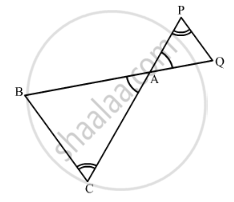Share
Notifications

View all notifications
Books Shortlist
Your shortlist is empty

# Solution for In the Following Figure, δAcb ~ δApq. If Bc = 8 Cm, Pq = 4 Cm, Ba = 6.5 Cm and Ap = 2.8 Cm, Find Ca and Aq. - CBSE Class 10 - Mathematics

Login
Create free account

Forgot password?

#### Question

In figure below ΔACB ~ ΔAPQ. If BC = 10 cm, PQ = 5 cm, BA = 6.5 cm and AP = 2.8 cm,
find CA and AQ. Also, find the area (ΔACB): area (ΔAPQ)#### Solution

We have,

ΔACB ~ ΔAPQ

Then,

"AC"/"AP"="CB"/"PQ"="AB"/"AQ"                   [Corresponding parts of similar Δ are proportional]

rArr"AC"/2.8=10/5=6.5/"AQ"

rArr"AC"/2.8=10/5 and 10/5=6.5/"AQ"

rArr"AC"=10/5xx2.8 and "AQ"=6.5xx5/10

⇒ AC = 5.6 cm and AQ = 3.25 cm

By area of similar triangle theorem

("Area"(triangleACB))/("Area"(triangleAPQ))/"BC"^2/"PQ"^2

=10^2/5^2

=100/25

= 4

Is there an error in this question or solution?

#### Video TutorialsVIEW ALL 

Solution In the Following Figure, δAcb ~ δApq. If Bc = 8 Cm, Pq = 4 Cm, Ba = 6.5 Cm and Ap = 2.8 Cm, Find Ca and Aq. Concept: Areas of Similar Triangles.
S